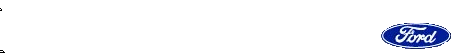Clarke's 96 SS Check Digit Verification To determine check digit (9th digit) of your car you need to take the VIN and assign any letters in the VIN with a set value (number) as follows: A = 1, B = 2, C = 3, D = 4, E = 5, F = 6, G = 7, H = 8, J = 1, K = 2, L = 3, M = 4, N = 5, O = 6, P = 7, R = 9, S = 2, T = 3, U = 4, V = 5, W = 6, X = 7, Y = 8, Z = 9 Any numbers in the VIN remain the same (not replaced) and thus you have a 17 digit number. Then you must multiply each of the 17 digits by a set "weight" (multiplier). The weight applied to each digit is as follows: 1st = 8, 2nd = 7, 3rd = 6, 4th = 5, 5th = 4, 6th = 3, 7th = 2, 8th = 10, 9th = 0 (check digit), 10th = 9, 11th = 8, 12th = 7, 13th = 6, 14th = 5, 15th = 4, 16th = 3, 17th = 2 After multiplying each VIN digit by the associated weight - add up the results of all 17 products. Then divide by 11 and the remainder is your check digit. If the remained is 10, then the check digit is 'X'. Here's an example: VIN = 1G1BL52P7TR115520 (note '7' is the check digit) VIN 1 G 1 B L 5 2 P 7 T R 1 1 5 5 2 0 Assigned Values 1 7 1 2 3 5 2 7 7 3 9 1 1 5 5 2 0 Multiplier 8 7 6 5 4 3 2 10 0 9 8 7 6 5 4 3 2 Add the products: (1 X 8) + (7 X 7) + (1 X 6) ... (0 X 2) = 337 Divide 337 by 11 which gives you 30, and a remainder of 7 which is the same as the 9th digit of the VIN.SS #1593